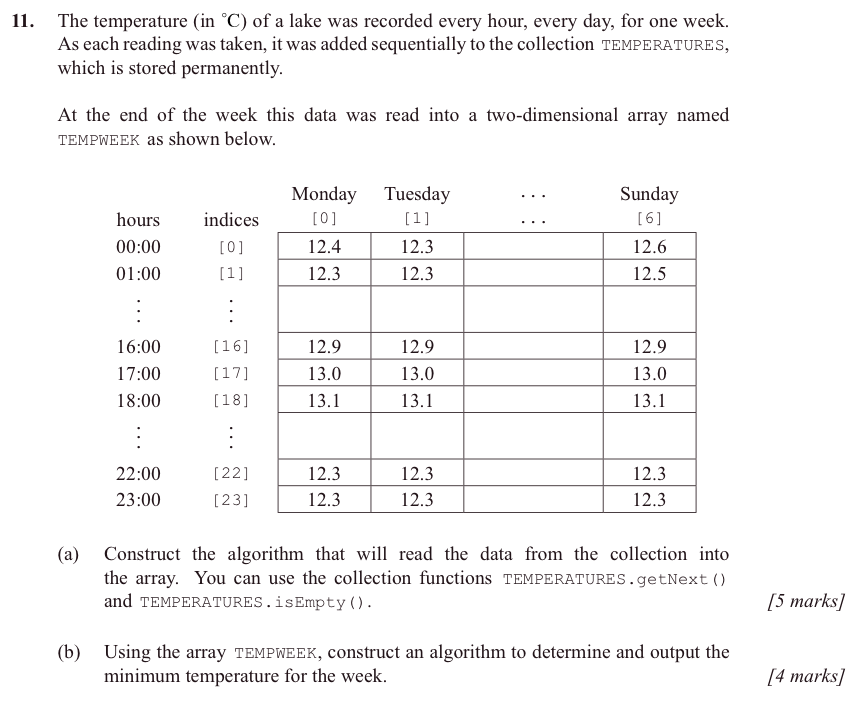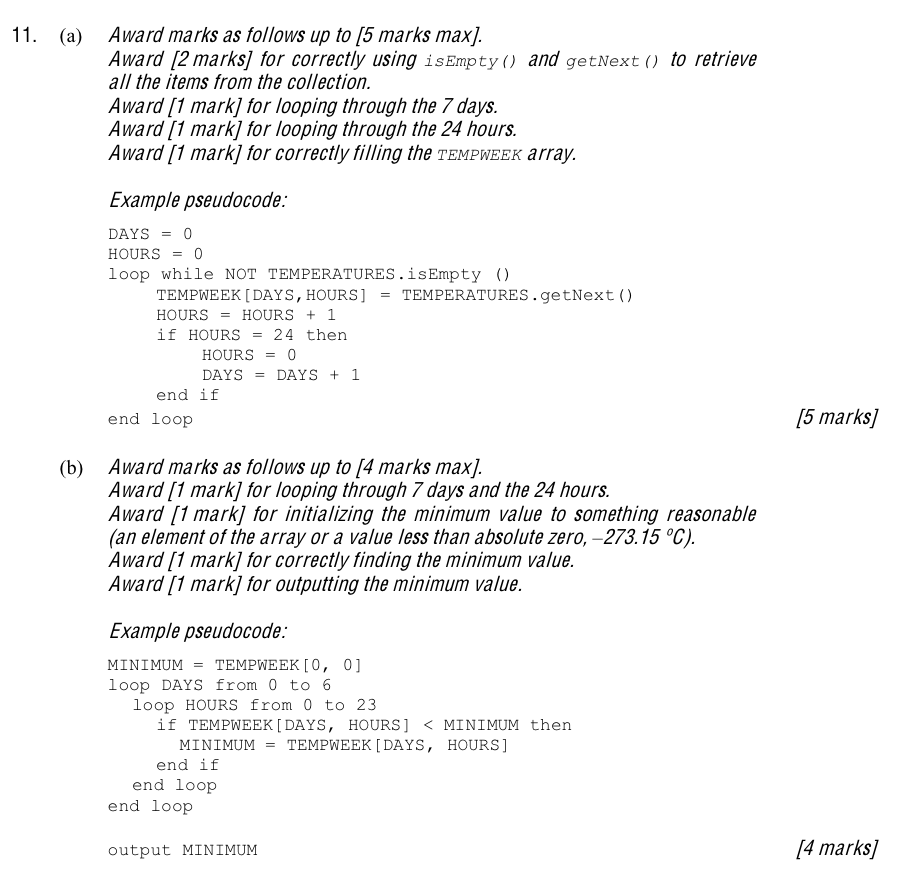Logout

5.1.5

Construct algorithms using two-dimensional arrays.

Teaching Note:

Sample Question:

From Sample Paper 1 - 2014:JSR Notes:

### Loops Within Loops & 2D Arrays

Review of one-dimentional arrays

First a vvvvvery quick summary of one-dimensional arrays:

int [] myIntArray = new int;

The above line makes an 32-bit object/reference variable called myIntArray which points to three contiguous 32-bit groups in memory which will be three ints. So then, we could go:

myIntArray = 23;
myIntArray = 88;
myIntArray = 99;

which assigns those values to the three elements of the array.

Review of the "old fashioned" braces way of declaring arrays

To better appreciate two dimentional arrays, it will be helpful to look at the way of explicitly declaring arrays. Here's that way that works, for a single dimension array, using the same one as above.

int [] myIntArray = { 23, 88, 99 };

So the declaration and the assignment all happen in the same line. There is no need to have a part of the declaration which stipulates the number of elements and reserves memory with the new operator, since the elements are all assigned right within the line.

At this point it will be good to note that the length of this array is 3, and we get that value with: myIntArray.length

### Now on to 2D Arrays, and their declaration

So what about a 2D array declared this old fashioned braces way?

In fact, a 2D array is an array of arrays, so the braces way of declaring it would be very straight-forward and as follows:

int [][] myIntArray = { { 23, 88, 99 } , { 55, 23, 46 } , { 36, 40, 73} , { 54, 93, 10 } };

Above, you have an array of four arrays; each one of those "sub"-arrays is three ints.
Do note that declaring it as 2D, we need to have two pairs of array declaration brackets: [][]

So what about the length of the above array? Well it itself is 4; it is an array of 4 sub-arrays, so its length is 4. We just use the length attribute as usual, as follows:

myIntArray.length

But when it comes time to loop through the entire array, we are going to want to know how many times to loop within each sub-array, so we'll want the sub-arrays' lengths too. Since the sub-arrays are indeed arrays, we can use the .length attribute for them too, but how to access that? Well, we take any one of the sub arrays, and get its .length attribute. To do that, we can use any of the sub-arrays, the th, or the st, or the nd, or the rd, it doesn't matter, since they are all the same length - 3. Here is the line for doing this:

myIntArray.length <----------

(Usually we just use the th, since all arrays, even one with only one element, will have a th element.)

### Loop Within Loop - "Nested Loops"

To access all the elements of a 2D array, we will be looping through the array of arrays; i.e. performing a nested looping situation. So let's first look at a straight-forward loop within a loop situation.

Consider the following nested looping situation (which for now is not of a 2d array):

***Use step through debugging with Netbeans or IntelliJ***

```        for (int i = 0; i < 4; i++) {
for (int k = 0; k < 3; k++) {
System.out.print(k + " - ");
}
System.out.println();
}```

The output would be:

0 - 1 - 2 -
0 - 1 - 2 -
0 - 1 - 2 -
0 - 1 - 2 -

Note that 0 1 and 2 go on the same line, since they are output with print( ), rather than println( ).

And the line by line trace of i and k would be:

i: 0
k: 0
print k, so 0 -
k++ to: 1
print k, so 0 - 1 -
k++ to: 2
print k, so 0 - 1 - 2 -
k ++ to 3, and k < 3 is no longer true, so exit the inner loop
print to a new line

i++ so,
i: 1
k: 0
print k, so 0 -
k++ to: 1
print k, so 0 -1 -
k++ to: 2
print k, so 0 - 1 - 2 -
k++ to 3, and k < 3 is no longer true, so exit the inner loop
print to a new line

i++ so,
i: 2
k: 0
print k, so 0 -
k++ to: 1
print k, so 0 - 1 -
k++ to: 2
print k, so 0 - 1 - 2 -
k++ to 3, and k < 3 is no longer true, so exit the inner loop
print to a new line

i++ so,
i: 3
k: 0
print k, so 0 -
k++ to: 1
print k, so 0 - 1 -
k++ to: 2
print k, so 0 - 1 - 2 -
k++ to 3, and k < 3 is no longer true, so exit the inner loop
print to a new line

i ++ to 4, and i < 4 is no longer true, so exit the outer loop

### Looping Throgh a 2D Array

So for each of the inner arrays, in turn, we will loop through them entirly, before moving onto the next inner array.

So... how do we loop through a 2D array? Well it's a loop within a loop, just like above. In the case of looping through a 2d array, the outer loop is controlled by the number of sub-arrays, and the inner loop is controlled by the number of elements in the sub-arrays. First, here's the code:

***Use step through debugging with Netbeans or IntelliJ***

```int [][] twoDArray = { { 55, 56, 57, 58 }, {22, 23, 24, 25} , {36, 37, 38, 39} };

for(int row = 0; row < twoDArray.length ; row++){
for(int col = 0; col < twoDArray.length; col++){
System.out.print(twoDArray[row][col]);
}
System.out.println("");
}```

Note the convention to name the loop control variables row and col, since this is what they represent if we are to think of our 2D array as a table.

By using .length for the one dimension, and .length for the other dimension, we can loop through the whole two dimensional structure, one element at at time.

The Output:

55 56 57 58
22 23 24 25
36 37 38 39

And the line by line trace of row and col would be:

row: 0
col++ to: 0
print [row][col], so , so 55
col++ to: 1
print [row][col], so , so 55 56
col++ to: 2
print [row][col], so , so 55 56 57
col++ to: 3
print [row][col], so , so 55 56 57 58
col++ to 4, and col < twoDArray.length, is no longer true, so exit the inner loop
print to a new line

row++ so,
row: 1
col++ to: 0
print [row][col], so , so 22
col++ to: 1
print [row][col], so , so 22 23
col++ to: 2
print [row][col], so , so 22 23 24
col++ to: 3
print [row][col], so , so 22 23 24 25
col++ to 4, and col < twoDArray.length, is no longer true, so exit the inner loop
print to a new line

row++ so,
row: 2
col++ to: 0
print [row][col], so , so 36
col++ to: 1
print [row][col], so , so 36 37
col++ to: 2
print [row][col], so , so 36 37 38
col++ to: 3
print [row][col], so , so 36 37 38 39
col++ to 4, and col < twoDArray.length, is no longer true, so exit the inner loop
print to a new line

row++ to row: 3, and row < twoDArray.length is no longer true, so exit the outer loop

### The Non-braces Way to Declare & Loop Through 2D Arrays

Declaring:

We declare a 2d array the same way as a single-dimension array, except that there are two dimensions, so two [] on either side of the assignment operator:

Example 1, an array to keep track of 10 row and 5 columns of random ints:

`int [][] my2DArray = new int ;`

or

Example 2, an array of 70 groups of related String values:

`String [][] s = new String ;`

### Assigning:

To assign data to 2d arrays, there are generally two approaches:
Most often we use the same loop within loop process as seen above, with the outer loop the rows, and so often using "row" as the loop control variable, and the inner loop being the columns, and so often using "col" as that loop control variable.

```for(int row = 0; row < my2DArray.length; row++){
for(int col = 0; col < my2DArray.length; col++){
my2DArray[row][col] = (int) (Math.random()*60);
}
}```

But often there is another approach, which would probably be used for the second example above. In this case, we don't loop through the items in each row with a for loop, rather, we just directly assign to the th element, and then the st element directly, with individual lines, over and over again. Though this isn't "looping" in the traditional for loop sense, it still accomplishes looping per se.

This way of doing things is often done in our GUI code workig with tables.

```for(int row = 0; row < myTable.length; row++){
System.out.println("What is the next student name you wish to enter?");
System.out.println("What is the address of this student?);